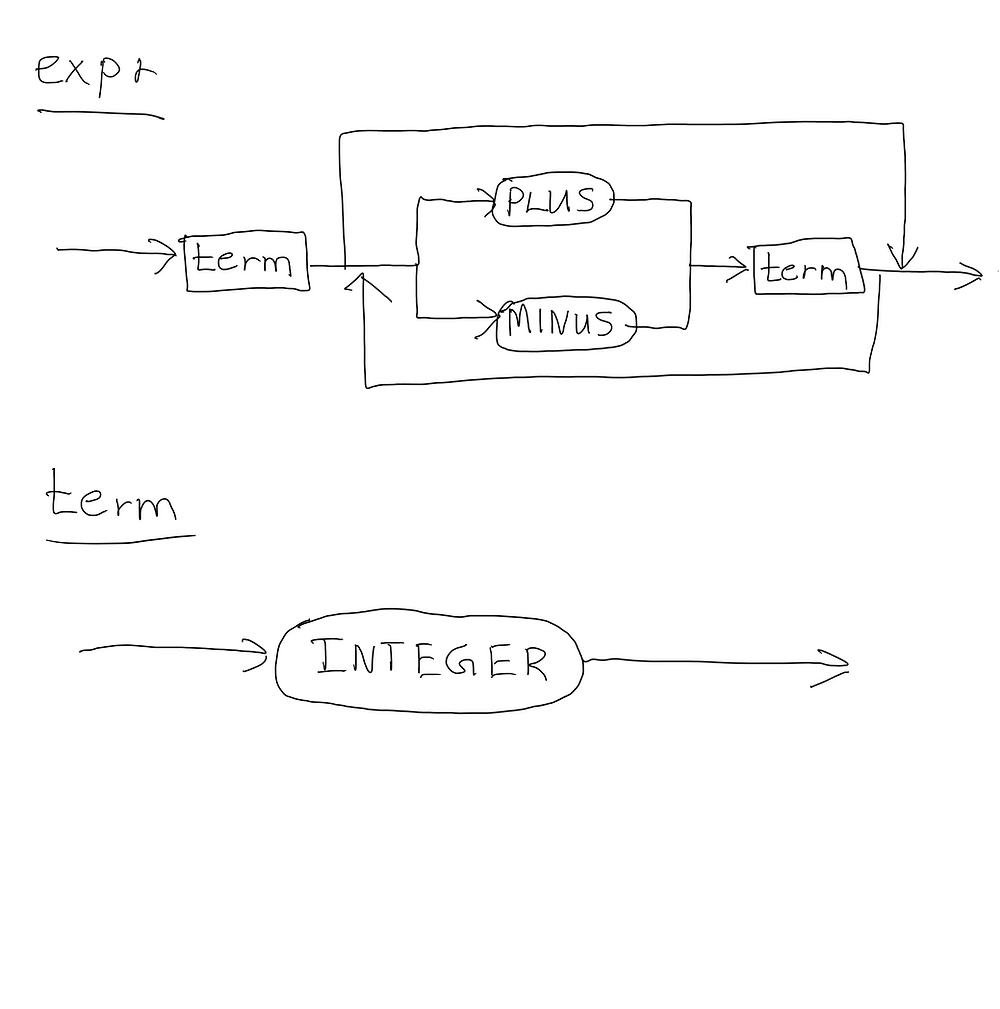Search
•Savidu Dias

# Developing a Simple Programming Language - Part 3: Syntax Diagrams

Oh hi there! I’ve been expecting you. Congratulations on making it to part 3 of Developing a Simple Programming Language. Today’s chapter is going to be short and sweet. In this chapter, we will develop our calculator further to be able to parse, and interpret arithmetic expressions that can have any number of addition and subtraction operations. For example, after this chapter, we will be able to do operations like “2 +3 - 4 + 5 - 6 + 7”. Before we get into that, let’s do a quick recap on what we have done so far.

In the last chapter, we discussed that an interpreter has three main components:

1. Tokens: Represent the smallest units of the source code.

2. Lexer: Converts the source code into a stream of tokens.

3. Parser: Relates the token stream into a structure.

The parser first attempts to identify a predefined structure in the stream of tokens. If we consider what we have done so far, the parser tries to identify a structure of INTEGER -> PLUS -> INTEGER, or INTEGER -> MINUS -> INTEGER.

Consider a situation where we have an input of “2 + 3”. The Parser first tries to see if the first token in our input is of type INTEGER, by communicating with the Lexer. Since the first token “2” is an integer, the lexer confirms that the structure holds true so far. Then, the Parser sees if the next token is either PLUS or MINUS with the Lexer. Finally, it checks if the last token is an integer, and gets a confirmation from the Lexer. Once confirmed, the parser adds 2 and 3 to produce the output 5.

Let’s get cracking with today’s work. As complicated as it may seem, what we have to do today is actually pretty simple. Since we can theoretically do additions and subtractions an infinite number of times, the best approach that we could use is to use a while loop in our parser to check if the current token is a PLUS, or a MINUS. If the condition no longer holds true, we can break out of the loop, and return the calculated result. All we have to do is add this to our Parser.

```/*
Returns the value of an INTEGER token
*/
int term() {
Token token = lexer.getCurrentToken();
lexer.eat(Type.INTEGER);
return (int) token.getValue();
}

int expr() {
int result = term();
Token currentToken = lexer.getCurrentToken();
while (currentToken.getType() == Type.PLUS || currentToken.getType() == Type.MINUS) {
if (currentToken.getType() == Type.PLUS) {
lexer.eat(Type.PLUS);
result = result + term();
} else if (currentToken.getType() == Type.MINUS) {
lexer.eat(Type.MINUS);
result = result - term();
}
currentToken = lexer.getCurrentToken();
}
return result;
}```

The term() function simply validates that the current token is an integer, and then returns its value. Let’s run our updated calculator and see if it works!

```\$ javac -d . Token.java
\$ javac -d . Lexer.java
\$ javac -d . Parser.java
\$ javac -d . Main.java
\$ java Main
calc> 1 + 6
7
calc> 2 + 4 - 6
0
calc> 3 + 8 - 9 + 11
13```

Congratulations! Our calculator can now do as many additions and subtractions in a single arithmetic expression. Before I let you go, let me show you a great way to design a programming language.

Let’s take a look at our calculator. A user’s input is interpreted by the expr() method like this:Wouldn’t it be easier if there was a better way to represent this? Well, worry no further, kids! I’m going to introduce you to an awesome way to graphically represent our calculator.What you see here is called a syntax diagram. A syntax diagram is a graphical representation of a programming language’s syntax. Our interpreter starts off by calling expr. Within expr is a term, followed by a PLUS, or MINUS token, followed by another term. This process could be looped through several times before finally exiting, and returning the result. This is a handy diagram that can show us which statements are allowed in our programming language, and which ones are not.

Syntax diagrams are pretty easy to understand. All we have to do is to simply follow the paths shown by the arrows, which may lead us to choices, or loops. If you haven’t figured it out already, the rectangular boxes point us to another syntax diagram, which the oval shapes represent Tokens.

If you’re interested in seeing what the Syntax diagrams for the programming language I developed as my College project looked like, check out what the programming language looks like, and how it was represented using syntax diagrams. If you feel overwhelmed after seeing that, don’t worry, it’s not as hard as you think! In fact, what we will end up making at the end of this tutorial series will also look like this.

To recap on Syntax Diagrams, they serve two main purposes:

1. Graphically representing the syntax of a programming language.

2. Directing us on how we should write our parser: the diagram can simply be mapped to our code.

Congratulations! We have now made a calculator that can do as many additions and subtractions as we want. In the next chapter, we will have our calculator do all sorts of complicated stuff, like multiplications, and divisions. As always, you’re welcome to try it yourself without looking at the next chapter. I’ll see you on the other side. Until then, peace out.

Previous Chapter: Part 2: Components of an Interpreter

Next Chapter: Part 4: Associativity, Precedence, and Grammars